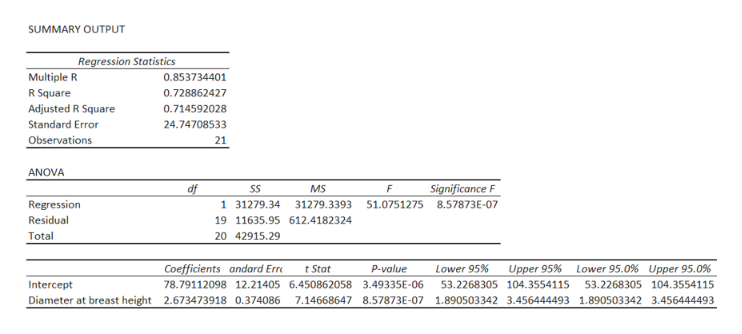# Measuring the height of a California redwood tree is very difficult because these trees grow to heights of over 300 feet. People familiar with these trees understand that the height of a California redwood tree is related to other characteristics of the tree, including the diameter of the tree at the breast height of a person. The data in the table represent the height (in feet) and diameter (in inches) at the breast height of a person for a sample of 21 California redwood trees.Assuming a linear relationship, use the least- squares method to compute the regression coefficients b0 and b1. State the regression equation that predicts the height of a tree based on the tree’s diameter at breast height of a person.Interpret the meaning of the slope in this equation.Predict the mean height for a tree that has a breast height diameter of 25 inches.HeightDiameter at breast heightBark thickness122.0201.1193.5362.8166.5182.082.0101.2133.5212.0156.0291.4172.5511.881.0111.1148.0262.5113.0121.584.0131.4164.0402.3203.3522.0174.0302.5159.0223.0205.0422.6223.5454.3195.0544.0232.5392.2190.5363.5100.081.4

Question
90 views

Measuring the height of a California redwood tree is very difficult because these trees grow to heights of over 300 feet. People familiar with these trees understand that the height of a California redwood tree is related to other characteristics of the tree, including the diameter of the tree at the breast height of a person. The data in the table represent the height (in feet) and diameter (in inches) at the breast height of a person for a sample of 21 California redwood trees.

1. Assuming a linear relationship, use the least- squares method to compute the regression coefficients b0 and b1. State the regression equation that predicts the height of a tree based on the tree’s diameter at breast height of a person.
2. Interpret the meaning of the slope in this equation.
3. Predict the mean height for a tree that has a breast height diameter of 25 inches.
 Height Diameter at breast height Bark thickness 122.0 20 1.1 193.5 36 2.8 166.5 18 2.0 82.0 10 1.2 133.5 21 2.0 156.0 29 1.4 172.5 51 1.8 81.0 11 1.1 148.0 26 2.5 113.0 12 1.5 84.0 13 1.4 164.0 40 2.3 203.3 52 2.0 174.0 30 2.5 159.0 22 3.0 205.0 42 2.6 223.5 45 4.3 195.0 54 4.0 232.5 39 2.2 190.5 36 3.5 100.0 8 1.4
check_circle

Step 1

1.

Step-by-step procedure to obtain the regression equation using Excel:

• In Excel sheet, enter Height in one column and enter Diameter at breast height in one column.
• In Data, select Data Analysis and choose Regression.
• In Input Variable y Range, select Height.
• In Input Variable x Range, select Diameter at breast height.
• Click Labels.
• Click Ok.

Output obtained using Excel is given below:help_outlineImage TranscriptioncloseSUMMARY OUTPUT Regression Statistics Multiple R 0.853734401 R Square 0.728862427 Adjusted R Square 0.714592028 Standard Error 24.74708533 Observations 21 ANOVA df MS Significance F SS F Regression 1 31279.34 31279.3393 51.0751275 8.57873E-07 Residual 19 11635.95 612.4182324 Total 20 42915.29 Upper 95.0 % Coefficients andard Err P-value Lower 95% Lower 95.0% t Stat Upper 95% 78.79112098 12.21405 6.450862058 3.49335E-06 53.2268305 104.3554115 53.2268305 104.3554115 Intercept Diameter at breast height 2.673473918 0.374086 7.14668647 8.57873E-07 1.890503342 3.456444493 1.890503342 3.456444493 fullscreen
Step 2

From the output, the regre...

### Want to see the full answer?

See Solution

#### Want to see this answer and more?

Solutions are written by subject experts who are available 24/7. Questions are typically answered within 1 hour.*

See Solution
*Response times may vary by subject and question.
Tagged in

### Statistics